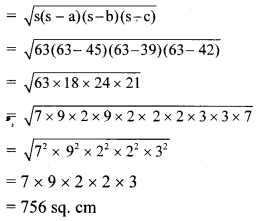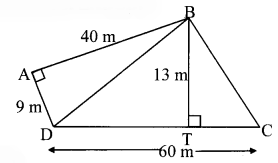# Maharashtra Board 8th Class Maths Practice Set 15.4 Solutions Chapter 15 Area

Balbharti Maharashtra State Board Class 8 Maths Solutions covers the Practice Set 15.4 8th Std Maths Answers Solutions Chapter 15 Area.

## Practice Set 15.4 8th Std Maths Answers Chapter 15 Area

Question 1.
Sides of a triangle are 45 cm, 39 cm and 42 cm, find its area.
Solution:
Sides of a triangle are 45 cm, 39 cm and 42 cm.
Here, a = 45cm, b = 39cm, c = 42cm
Semi perimeter of triangle = s = $$\frac { 1 }{ 2 }(a+b+c)$$
= $$\frac { 1 }{ 2 }(45+39+42)$$
= $$\frac { 126 }{ 2 }$$
= 63
Area of a triangle∴ The area of the triangle is 756 sq.cm.

Question 2.
Look at the measures shown in the given figure and find the area of ☐PQRS.Solution:
A (☐PQRS) = A(∆PSR) + A(∆PQR)
In ∆PSR, l(PS) = 36 m, l(SR) = 15 m
A(∆PSR)
= $$\frac { 1 }{ 2 }$$ x product of sides forming the right angle
= $$\frac { 1 }{ 2 }$$ x l(SR) x l(PS)
= $$\frac { 1 }{ 2 }$$ x 15 x 36
= 270 sq.m
In ∆PSR, m∠PSR = 90°
[l(PR)]² = [l(PS)]² + [l(SR)]²
…[Pythagoras theorem]
= (36)² + (15)²
= 1296 + 225
∴ l(PR)² = 1521
∴ l(PR) = 39m
…[Taking square root of both sides]
In ∆PQR, a = 56m, b = 25m, c = 39mA(☐PQRS) = A(∆PSR) + A(∆PQR)
= 270 + 420
= 690 sq. m
∴ The area of ☐PQRS is 690 sq.m

Question 3.
Some measures are given in the figure, find the area of ☐ABCD.Solution:
A(∆BAD) = $$\frac { 1 }{ 2 }$$ x product of sides forming the right angle
= $$\frac { 1 }{ 2 }$$ x l(AB) x l(AD)
= $$\frac { 1 }{ 2 }$$ x 40 x 9
= 180 sq. m
In ∆BDC, l(BT) = 13m, l(CD) = 60m
A(∆BDC) = $$\frac { 1 }{ 2 }$$ x base x height
= $$\frac { 1 }{ 2 }$$ x l(CD) x l(BT)
= $$\frac { 1 }{ 2 }$$ x 60 x 13
= 390 sq. m
A (☐ABCD) = A(∆BAD) + A(∆BDC)
= 180 + 390
= 570 sq. m
∴ The area of ☐ABCD is 570 sq.m.

Scroll to Top